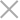# P1358 扑克牌

• 作者: 胸口碎大石6260688
• 来源: 51数据库
• 2021-07-10

## 输入输出样例

5 2
3 1


20


20 19
1 1 1 1 1 1 1 1 1 1 1 1 1 1 1 1 1 1 1


8707


## 说明/提示

代码如下：

#include<iostream>
#include<cstdio>
using namespace std;
const int maxm=110;
int n,m,sum=1;
int a[maxm],f[maxm];
int main(){
scanf("%d%d",&n,&m);
for(int i=1;i<=m;i++) scanf("%d",&a[i]);
f=1;
f=1;f=1;
for(int i=2;i<=n;i++){
f[i]=1;
for(int j=1;j<=min(i,100);j++){//m最大为100，也就是最多用到第100列的值
f[i][j]=(f[i-1][j-1]+f[i-1][j])%10007;
//	cout<<f[i][j]<<" ";
}
//	cout<<endl;

}
for(int i=1;i<=m;i++){
//	cout<<sum<<" ";
sum=(sum*f[n][a[i]])%10007;
//	cout<<f[n][a[i]]<<endl;
n-=a[i];
}
printf("%d\n",sum);
return 0;
}


Java相关